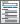Home  /  Products  /  Features  /  Tests, predictions, and effects

## Tests, predictions, and effects

Hypothesis testing

Generalized testing• Ability to combine separate estimates into one combined estimate
• Robust covariance matrix of combined estimates
• Tests of linear and nonlinear combinations of estimates across fitted models
• Point estimates and confidence intervals of linear and nonlinear combinations of estimates across fitted models
• Ability to obtain predicted values after all estimation commands
• Predictor types that are tightly coupled to the estimation command
• Default predicted value that is most relevant to the fitted model

Generalized predictions• Linear and nonlinear combinations of
• Standard predictions
• Equation index values
• Estimated coefficients
• Data
• Inferential statistics for generalized predictions:
• Point estimates
• Standard errors
• Variances
• Wald test statistics
• Significance levels
• Pointwise confidence intervals

Postestimation statistics

Linear and nonlinear combinations of coefficients• Point estimates
• Standard errors
• Confidence intervals
• Tests of significance
• Covariances of transformations
• Support for survey and clustered data
• Comparison of means
• Cohen's d
• Hedge's g
• Glass's Δ
• Point/biserial correlation
• Confidence intervals
• Variance explained by regression and ANOVA
• Eta-squared—η2
• Omega-squared—ω2
• Confidence intervals

Watch Introduction to margins in Stata tutorials
Watch Profile plots and interaction plots in Stata tutorials
• View and run all postestimation features for your command
• Automatically updated as estimation commands are run
• Combine results from multiple estimation commands
• Specify identities and declare exogenous variables
• Obtain dynamic and static forecasts• Use simulation methods to obtain prediciction intervals
• Specify alternative scenarios and perform "what-if" analyses

Specification link test for single-equation modelsSave and restore estimation resultsBayesian postestimation